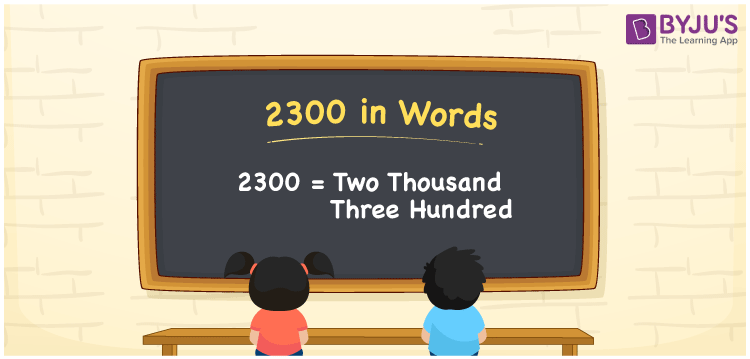# 2300 in Words

The number 2300 in words is Two thousand three hundred. The number 2300 can easily be converted to words by using the place value of a specific number. As a result, a place value table is necessary to create the number names. For example, if you spent Rs. 2300 for purchasing a book, you may write “I spent Rs. Two thousand three hundred for purchasing a book”. We’ll learn how to spell 2300 in English and how to write the number name using the place value chart in this article.

 2300 in Words: Two Thousand Three Hundred. Two Thousand Three Hundred in Numerical Form: 2300.

## 2300 in English Words## How to Write 2300 in Words?

For converting the numbers to words, we can use a place value chart. Below is the place value chart for the number 2300.

 Thousands Hundreds Tens Ones 2 3 0 0

Here, the expanded form of the number 2300 is given.

= 2 × Thousand + 3 × Hundred + 0 × Ten + 0 × One

= 2 × 1000 + 3 × 100

= 2000 + 300

= 2300

= Two thousand three hundred

Hence, 2300 in words is two thousand three hundred.

The number 2300 is a natural number that comes after 2299 and before 2301.

2300 in words – Two thousand three hundred

Is 2300 an odd number? – No

Is 2300 an even number? – Yes

Is 2300 a perfect square number? – No

Is 2300 a perfect cube number? – No

Is 2300 a prime number? – No

Is 2300 a composite number? – Yes

## Frequently Asked Questions on 2300 in Words

Q1

### Write 2300 in words.

2300 in words is two thousand three hundred.

Q2

### Simplify 2500 – 200, and express in words.

Simplifying 2500 – 200, we get 2300. Hence, 2300 in words is two thousand three hundred.

Q3

### How to write two thousand three hundred into numbers?

Two thousand three hundred in numbers is 2300